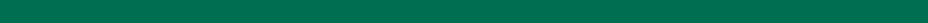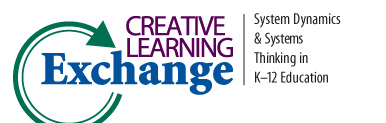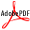>
CLE Curriculum Search
Search results for: Social Studies
112 records found. Currently displaying page 5 of 12 [<< Prev] 1 2 3 4 5 6 7 8 9 10 11 12 [Next >>]
 Lessons from The Lorax: Using Graphs to Study Change Author(s): Rob Quaden, & Alan Ticotsky Subject: Cross-Curricular In this lesson, students read The Lorax and draw graphs to illustrate the changes that happen over the course of the story. Using simply stated questions, readers grapple with the complex themes in the book and movie. Students will investigate how cycles compete for dominance, and think about how the needs of business and natural resources can collide. Complex Systems Connection: Separate Cause and Effect, Short and Long Term Conflicts. Short-term focus on making money results in depletion of resource and environmental degradation over time and the collapse of the business. Actions and consequences are separated by time.PDF Lessons in Mathematics Section 0: Overview Author(s): Diana M. Fisher Subject: Math This book provides a set of tools that enables educators to teach mathematics using the framework of System Dynamics. Section 0 covers basic skills in representing equations using a visual modeling tool, the STELLA software. Available from iseesystems. More about the book at: http://www.iseesystems.com/store/college_university/MathBook.aspx Lessons in Mathematics Section 1: Linear Behavior Author(s): Diana M. Fisher Subject: Math This book provides a set of tools that enables educators to teach mathematics using the framework of System Dynamics. Section 1 on linear motion covers the theory of finite differences and distance-time and position-time exercises using a motion detector. More about the book at: http://www.iseesystems.com/store/college_university/MathBook.aspx Lessons in Mathematics Section 2: Quadratic Behavior Author(s): Diana M. Fisher Subject: Math This book provides a set of tools that enables educators to teach mathematics using the framework of System Dynamics. Section 2 on quadratic behavior covers the theory of finite differences and distance-time and position-time exercises using a motion dete More about the book at: http://www.iseesystems.com/store/college_university/MathBook.aspx Lessons in Mathematics Section 3: Exponential Behavior Author(s): Diana M. Fisher Subject: Math This book provides a set of tools that enables educators to teach mathematics using the framework of System Dynamics. Section 3 covers the theory of finite differences for exponential functions. Students build models of many processes, including bacteria More about the book at: http://www.iseesystems.com/store/college_university/MathBook.aspx Lessons in Mathematics Section 4: Review Author(s): Diana M. Fisher Subject: Math This book provides a set of tools that enables educators to teach mathematics using the framework of System Dynamics. Section 4 reviews previous concepts, combines model structures to solve new problems and extends problems from previous lessons. Availab More about the book at: http://www.iseesystems.com/store/college_university/MathBook.aspx Lessons in Mathematics Section 5: Oscillatory Behavior Author(s): Diana M. Fisher Subject: Math This book provides a set of tools that enables educators to teach mathematics using the framework of System Dynamics. Section 5 covers sinusoidal functions, including simple harmonic motion. Distance versus time lessons using a motion detector are also inPDFMore about the book at: http://www.iseesystems.com/store/college_university/MathBook.aspx Lessons in Mathematics Section 6: Convergent and Logistic Behavior Author(s): Diana M. Fisher Subject: Math This book provides a set of tools that enables educators to teach mathematics using the framework of System Dynamics. Section 6 takes students through the steps from exponential to convergent to logistic models, culminating with an application to a systemPDFMore about the book at: http://www.iseesystems.com/store/college_university/MathBook.aspx Lessons in Mathematics Section 7: Differential Equations Author(s): Diana M. Fisher Subject: Math This book provides a set of tools that enables educators to teach mathematics using the framework of System Dynamics. Section 7 builds on the skills of Sections 5 and 6 using classic problems studied with differential equations. Exponential, convergent anPDFMore about the book at: http://www.iseesystems.com/store/college_university/MathBook.aspx Lessons in Mathematics Section 8: Miscellaneous Topics Author(s): Diana M. Fisher Subject: Math This book provides a set of tools that enables educators to teach mathematics using the framework of System Dynamics. Section 8 presents a natural extension of linear and exponential growth study - arithmetic and geometric sequences. Application to an age More about the book at: http://www.iseesystems.com/store/college_university/MathBook.aspx
Sorted Ascending by Title Hide Descriptions